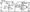# Analog Electronic Circuits June 2010

Note: 1. Answer any FIVE full questions, selecting at least TWO questions from each part.

2. Draw equivalent circuit wherever necessary.

PART-A

1 a. Explain the different diode equivalent circuits – with necessary approximations if any.

b.  Define clipper circuit. Draw and explain symmetrical double ended diode clipper circuit with the help of transfer characteristics.

c.   A full wave rectifier using centre tapped transformer supplies a resistive load of 1 K Q. The transformer secondary end to end voltage is 60 V rms at 50 Hz. The filer capacitance is 500 jj.F. Calculate : i) Ripple factor ; ii) Output resistance of the filter (Ro) ; iii) Vdc ; iv) Idc ; v) % regulation.

2  a. Explain Emitter bias circuit, with the help of B.E. loop and C.E. loop. Write the necessary equations.

b.  Explain the circuit of a transistor switch being used as an inverter

c.   Determine the dc bias voltage Vce and the current Ic for the voltage – divider configuration of Fig.2(c), show below.

3 a.  Define h – parameters. Draw the complete hybrid equivalent circuit of a transistor.

b.  Sketch the re – equivalent circuit of CE fixed bias configuration and derive the expression for Ar, Ai, Zi and Zo. Show the phase relationship between input and output wave form.

c.   For common base configuration shown in Fig.3(c). Determine :

i) re ;            ii) Zi ;                 iii)Zo ;            iv) Ar ;               v) Ai.4 a. Describe miller effect and derive an equation for miller input and output capacitances.

b.  Discuss the low frequency response of BJT amplifier and give expression for lower cut-off frequency due to Cc, Ce and Cs.

PART-B

5 a. Draw the cascade configuration and list the advantages of this circuit.

b.  With necessary equivalent circuit diagram obtain the expression for Zin, Zo and Av for a Darlington Emitter follower.

c.   Derive expression for Zif and Zof for voltage series feedback amplifier and list the advantages of negative feedback amplifier.

6 a. Give the definition of power amplifiers and list the types of power amplifier based on the location of 2-point.

b.  Explain the workings of class – B push pull amplifier. Obtain an expression for maximum conversion efficiency of this amplifier.

c.   Calculate the harmonic-distoration components for an output signal having fundamental amplitude of 2.5 V, second harmonic amplitude of 0.25, third harmonic amplitude of 0.1 V and fourth harmonic amplitude of 0.05 V and also calculate the total harmonic distoration for the amplitude components given above.

7 a. Explain how a feedback circuit can be used as Oscillators.

b.  Explain with help of circuit diagram, the working of an RC phase shift oscillator.

c.   A quartz crystal has L = 0.12 H, C ~ 0.04 pF, Gm = 1 pF and R = 9.2 K Q. Find:

i) Series resonant frequency;       ii) Parallel resonant frequency.

8 a. Discuss the difference between FET and BJT.

b.  With a necessary oc equivalent model of JFET common-drain configuration. Obtain the expression for Zi, Zo and Av.

c.   Explain FET small signal model with help of graphical representation of gm.